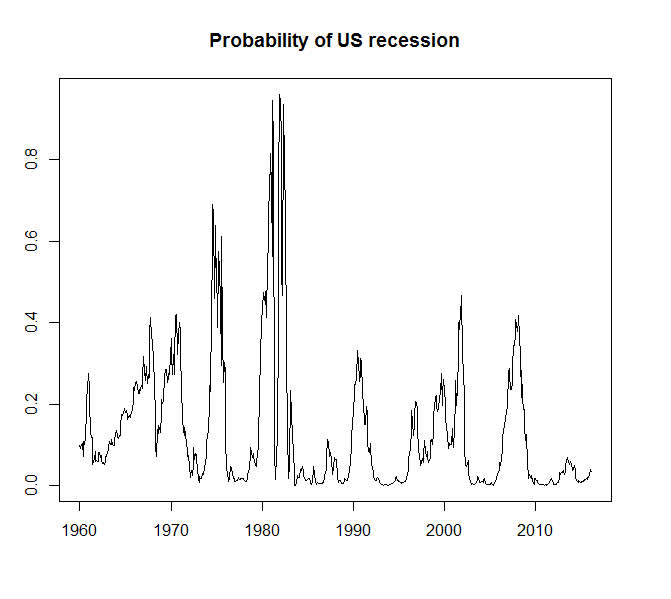Friday, 1 April 2016

Predicting US Recessions Using the Yield Curve

In last week’s Economic Forecasting and Analysis class, we talked about how the yield curve could be used to predict economic recessions. There is a deep literature on this topic going back to the late 1980s. The basic idea is that the market for government bonds is very sensitive to monetary policy. Normally, the yield curve is upward sloping, the difference between yields of long bonds (say 10 years) and short bonds (3 month T bills) is positive to compensate investors for the additional risk in holding bonds for longer periods of  time. In recessions, central banks cut benchmark interest rates in order to stimulate the economy. A reduction in interest rates lowers the yield on new issues of government bonds. Consequently, if the bond market expects a recession and a cut in central bank rates, bond investors will start selling short term bonds and buy long term bonds to lock in the higher yields. This pushes the price of long bonds up and reduces the price of short bonds. Since bond prices and yields are inversely related, short yields rise and long yields fall. The yield curve inverts when the yield spread becomes negative.

The Federal Reserve Bank of New York has been actively studying this topic for a long time, and there is lots of useful information posted on their website. The existing literature shows that the yield spread is a good predictor of recessions one year into the future.  The New York Fed posts data on the NBER dating of US recessions and the yield spread and uses this data to estimate the probability of a US recession using a probit model. The dependent variable is a binary variable equal to one if the economy is currently in a recession and zero otherwise. The independent variable is a 12 month lag of the yield spread. The New York Fed posts the data, model results, and recession probability chart but this is still a worthy exercise to work through. For estimation in R, I have loaded the data into a csv file with the spread variable lagged 12 months.

Here is the probit regression output.

```Deviance Residuals:
Min       1Q   Median       3Q      Max
-2.4163  -0.5152  -0.2630  -0.1147   2.8313

Coefficients:
Estimate Std. Error z value Pr(>|z|)
(Intercept) -0.54086    0.08047  -6.721  1.8e-11 ***
Spread      -0.65560    0.06702  -9.782  < 2e-16 ***
---
Signif. codes:
0 ‘***’ 0.001 ‘**’ 0.01 ‘*’ 0.05 ‘.’ 0.1 ‘ ’ 1

(Dispersion parameter for binomial family taken to be 1)

Null deviance: 540.93  on 673  degrees of freedom
Residual deviance: 398.29  on 672  degrees of freedom
AIC: 402.29

Number of Fisher Scoring iterations: 6
```

The estimated parameter values are close but not identical to the ones from the New York Fed (intercept = -0.5330, slope = -0.6330. Different estimation procedures for probit may be the result of the minor differences in results.

Here is the probability of US recession chart.Probabilities over 40% seem to be accurate predictors of future recessions.

The probit estimates can be used to predict the probability of a US recession. The following table shows the probabilities for the next 12 months. The probability of a US recession 12 months from now is currently very low at 6.6%.

```      recession prob 2016/2017
March     0.03154117         3
April     0.03596135         4
May       0.02441563         5
June      0.01899524         6
July      0.02056696         7
Aug       0.02757996         8
Sept      0.02556458         9
Oct       0.02972609        10
Nov       0.02595748        11
Dec       0.03154117        12
Jan       0.04087655         1
Feb       0.06621488         2```

The R script is posted below.

#########################################################
#  Economic forecasting and analysis
#  April 2016
#  Predicting US Recessions Using the Yield Curve
##########################################################

# model and data from:
# https://www.newyorkfed.org/research/capital_markets/ycfaq.html

View(Book1)

mydata = ts(Book1, start=1960, freq =12)

summary(myprobit)

prob_in = predict(myprobit,
type = "response")

plot(prob_in)

plot(df1[,2], main="Probability of US recession", ylab="", xlab="")

1.92,
2.18,
2.34,
2.29,
2.10,
2.15,
2.05,
2.14,
2.01,
1.83,
1.47

))

prob_out = predict(myprobit, newdata,
type = "response")

prob_out

prob_out_t = cbind(prob_out,c(seq(from = 3,to = 12, by=1),1,2))
colnames(prob_out_t)=c("recession prob", "2016/2017")

prob_out_t
rownames(prob_out_t) = c("March", "April","May", "June", "July","Aug", "Sept","Oct", "Nov", "Dec","Jan", "Feb" )
prob_out_t

1 comment:

1.eToro is the ultimate forex broker for new and established traders.